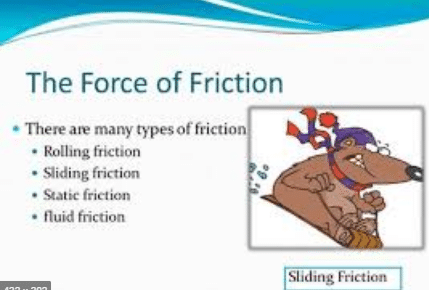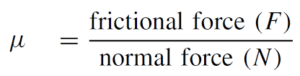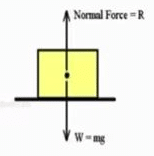Mechanics

# What are the Different Types of friction?Friction is the force that opposes the motion of moving objects. Basically, there are three types of fiction, Kinetic friction, Static friction and limiting friction. Some other Examples of friction are rolling friction, sliding friction and fluid friction. Frictional force occurs due to imperfections, which are mostly microscopic, between the surfaces in contact.
Now!

## What is friction?

Have you ever noticed why a moving ball stops? Why bicycle stops when the cyclist stops pedalling? Naturally, there must be some force that stops moving objects. Since a force not only moves an object but also stops moving object. Friction is a force that comes into action as soon as a body is pushed or pulled over a surface.

In the case of solids, the force of friction between two bodies depends upon many factors such as the nature of the two surfaces in contact and the pressing force between them. Rub your palm over different surfaces such as table, carpet, polished marble surface, brick, etc. You will find smoother is the surface, easier it is to move your palm over the surface. Moreover, harder you press your palm over the surface, more difficult would it be to move.

### Examples of friction in everyday lifeSome examples of friction in daily life will be listed below:

1. The wheels of a car that move on the pavement, intentionally high to give the driver greater control over the vehicle.
2. The design of all kinds of vehicles, be they submarines, boats and all those that move on water.
3. The friction between an aeroplane and the air, when it is flying. This friction is dependent on the aerodynamic design.
4. An object perched on the ground, which is very difficult to give the initial impulse but somewhat easier to move once that impulse has been produced.
5. Sliding a body on wet plastic, which gives it more travel than on dry plastic, precisely because water decreases the friction force between surfaces.
6. A match when it collides with the box, generating the combustion necessary to ignite.
7. Rubbing between two objects such as stones or wood, which generate heat and eventually fire.
8. An ice skating rink, where friction is reduced and therefore skates are used for greater displacement.
9. The sole of the shoes, with special engravings to increase friction.
10. Rub between both hands or any part of the body.

#### Why friction opposes motion?

No surface is perfectly smooth. A surface that appears smooth has pits and bumps that can be seen under a microscope. A magnified view of two smooth surfaces in contact shows the gaps and contacts between them. The contact points between the two surfaces form a sort of cold welds. These cold welds resist the surfaces from sliding over each other. Adding weight over the upper block increases the force pressing the surfaces together and hence,  increases the resistance. Thus, greater is the pressing force greater will be the friction between the sliding surfaces.

Actually, whenever the surface of one body slides over that of another, each body exerts a frictional force on the other. The frictional force on each body is in a direction opposite to its motion relative to the other body. Frictional forces automatically opposite this relative motion and never aid it. Even when there is no relative motion, frictional forces may exist between surfaces.

### Friction force formula

Fiction is equal to the applied force that tends to move a body at rest. It increases with the applied force. Friction can be increased to a certain maximum value. It does not increase beyond this.

### Coefficient of friction formula

The maximum value of friction is known as the force of limiting friction (Fs). It depends on the normal reaction (pressing force) between the two surfaces in contact. The ratio between the force of limiting friction Fs and the normal reaction R is constant. This constant is called the coefficient of friction and is represented by μ. ThusFS = μR

If m is the mass of the block, then for the horizontal surface:

R = mg

Hence

FS=μ mg

Table of the coefficient of friction between some common materials:

 Materials μs (coefficient of friction) Glass and Glass 0.9 Glass and Metal 0.5-0.7 Ice and Wood 0.05 Iron and Iron 1.0 Rubber and Concrete 0.6 Steel and Steel 0.8 Tyre and Road,dry 1 Tyre and Road ,wet 0.2 wood and metal 0.2 – 0.6

### Factors that affect friction

• Normal force:The force which is perpendicular to the surface is called the normal force. When one object moves on another object, the weight of the object acts downward and its reaction force known as a normal force acts upward according to newton’s third law.

The normal force sometimes called the loading force arises from the elastic properties of the bodies. Such bodies are never entirely rigid, and when a force is exerted on one body that is prevented from moving in the direction of the force, the body pushes back to oppose being stretched or deformed.

For a block resting on a horizontal table or sliding along it, the normal force is equal in magnitude to the weight of the block. Because the block has no vertical acceleration, the table must be exerting a force on the block that is directed upward and is equal in magnitude to the downward pull of the earth on the block, that is,  equal to the block’s weight.

• Material:

The material from which the two surfaces are made roughness of the surfaces in contact (coefficient of friction, μ).

### List of mainTypes of friction

• Static friction
• Kinetic friction
• Sliding friction
• Rolling friction
• Fluid friction
• Dry friction
• Lubricated friction
• Skin friction

Now we learn detail about friction types.

### Static friction

“The frictional forces acting between the surfaces at rest with respect to each other are called forces of static friction.”

Consider a block of mass m placed on a horizontal surface. The weight of the block is mg, which is balanced by normal force N ( reaction of the horizontal surface).Suppose a force F is applied on the resting block which is balanced by the equal and opposite force of static friction f. As force F increases, the force of static friction falso increases until freaches a certain maximum value just before the sliding the block. The direction of the static frictional force is along the contact surface and opposite in direction of any applied force. This force of static friction depends upon

• The normal force N
• The nature of the surfaces in contact

fs ≤ μ N

(fsmax =μ s N

#### Coefficient of static friction

μ s = (fs) max/N

Here (fsmax  is the maximum value of the force of static friction, just before the sliding or movement of the block is called the coefficient of static friction (μ s). It depends upon the nature of the surfaces in contact.

#### Examples of static friction

Here are some examples of static friction :

1. A heavy box against the ground, difficult to lift and move.
2. A nightstand resting on a light table.
3. A dry and a wet plastic, where the second has less friction than the first.
4. Friction toys that imitate the behaviour of force in the case of vehicles, but in a static way.
5. The rest of the body when a person leans against the wall.

### Kinetic friction

“The frictional forces acting between the surfaces in relative motion are called the force of kinetic friction.”

When the value of applied force F is greater than the maximum force of static friction (fsmax , then the block starts moving and has accelerated motion F > (fsmax.

Let the value of the applied force F is so adjusted that the block moves with uniform velocity v.In this case force of friction between the two surfaces is known as the force of kinetic friction f. This force depends upon:

• The normal force
• The nature of the surfaces

When the block is moving with uniform velocity then:

fN

It should be noted that:

(fsmax > fk

μs > μk

#### Coefficient of kinetic friction

Where μis called Coefficient of kinetic friction. It is defined as “The ratio of the magnitude of the force of kinetic friction to the magnitude of the normal force is called the coefficient of kinetic friction for the surfaces involved.”If fk represents the magnitude of the force of  kinetic friction, we can write as:

μ=fk/N

Where μk is the coefficient of static friction and N is the magnitude of the normal force. Both μs and μk are dimensionless constants, each being the ratio of the magnitudes of two forces. Usually, for a given pair of surfaces μs > μk.

#### Examples of Kinetic Friction

The following are examples of Kinetic friction :

1. Feet against the ground, when walking.
2. The wheels of a bicycle against the ground.
3. The friction between an aeroplane and the air.
4. Underwater vehicles, with the friction it exerts on water.
5. Skates on an ice or concrete rink.

### Sliding friction

When an object slides over another surface, the frictional force that comes into action is sliding friction.

### Sliding friction examples

Rubbing both hands with each other is the simple example of sliding friction.

### Fluid friction

“The frictional force of an object moving through a fluid like water or air is called fluid friction.”It is also called drag force.

### Rolling friction

The wheel is one of the most important inventions in the history of mankind. The first thing about a wheel is that it rolls as it moves rather than to slide. This greatly reduces friction. Why?

#### Rolling friction examples

When the axle of a wheel is pushed, the force of friction between the wheel and the ground at the point of contact provides the reaction force. The reaction force acts at the contact points of the wheel in a direction opposite to the applied force. The wheel rolls without rupturing the cold welds. That is why the rolling friction is extremely small than sliding friction. The fact that rolling friction is less than sliding friction is applied in ball bearings or roller bearings to reduce losses due to friction.

The wheel would not roll on pushing it if there would be no friction between the wheel and the ground. Thus, friction is desirable for wheels to roll over a surface. It is dangerous to drive on a wet road because of the friction between the road and the tyres are very small. This increases the chance of slipping the tyres from the road. The threading improves road grip and makes it safer to drive even on the wet road.

A cyclist applies brakes to stop the bicycle. As soon as brakes are applied, the wheels stop rolling and begin to slide over the road. Since sliding friction is much greater than rolling friction. Therefore, the cycle stops very quickly.

Braking and skidding:

The wheels of moving vehicles have two velocity components:

1. The motion of wheels along the road.
2. Rotation of wheels about their axis.

To move a vehicle on the road as well as to stop a moving vehicle requires friction between its tyres and the road. For example, if the road is slippery or the tyres are worn out then the tyres instead of rolling, slip over the road. The vehicle will not move if the wheels start slipping at the same point on the slippery road. Thus for the wheels to roll, the force of friction (gripping force) between the tyres and the road must be enough that prevents them from slipping.

Similarly to stop a car quickly, a large force of friction between the tyres and the road is needed. But there is a limit to this force of friction that tyres can provide. If the brakes are applied too strongly, the wheels of the car will lock up (stop turning) and the car will skid due to its large momentum. It will lose its directional control that may result in an accident. In order to reduce the chance of skidding, it is advisable not to apply brakes too hard that lock up their rolling motion especially at high speeds. Moreover, it is unsafe to drive a vehicle with worn-out tyres.

Sometimes friction is most desirable.

• We cannot write if there would be no friction between paper and a pencil.
• Friction enables us to walk on the ground.
• We cannot run on slippery ground.
• A slippery ground offers very little friction. Hence, anybody who tries to run on a slippery ground may meet an accident
• Similarly, it is dangerous to apply brakes with full force to stop a fast-moving vehicle on a slippery road.
• Birds could not fly if there is no air resistance. The reaction of pushed air enables the birds to fly.
• Thus in many situations, we need friction while in other situations we need to reduce it as much as possible.

• Friction is undesirable when moving at high speeds because it opposes the motion and thus limits the speed of moving objects.
• Most of our useful energy is lost as heat and sound due to the friction between various moving parts of machines.
• In machines, friction also causes wear and tear of their moving parts.

### Methods of reducing friction

The friction can be reduced by:

• Making the sliding surfaces smooth.
• Making the fast-moving objects a streamlined shape (fish shape) such as cars, aeroplanes, etc. This causes the smooth flow of air and thus minimizes air resistance at high speeds.
• Lubricating the sliding surfaces.
• Using ball bearings or roller bearings, because the rolling friction is lesser than the sliding friction.